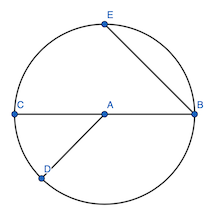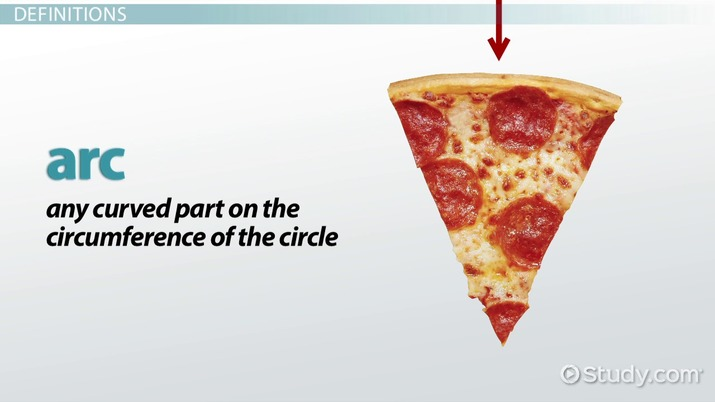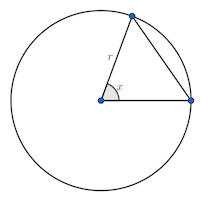# Area of a Segment of a Circle

Anderson Gomes Da Silva, Beverly Maitland-Frett
• Author
Anderson Gomes Da Silva

Anderson holds a Bachelor's and Master's Degrees (both in Mathematics) from the Fluminense Federal University and the Pontifical Catholic University of Rio de Janeiro, respectively. He was a Teaching Assistant at the University of Delaware (UD) for two and a half years, leading discussion and laboratory sessions of Calculus I, II and III. In the Winter of 2021 he was the sole instructor for one of the Calculus I sections at UD.

• Instructor
Beverly Maitland-Frett

Beverly has taught mathematics at the high school level and has a doctorate in teaching and learning.

Learn what a segment of a circle is. Discover the formula for finding the area of a segment of a circle, and identify theorems for segments of a circle. Updated: 11/16/2021

Show

## What is a Segment of a Circle?

Observe Figure 1. Given a point {eq}A {/eq}, a circumference is a set of all points that are at a fixed distance {eq}r {/eq} to {eq}A {/eq}, with the corresponding circle being the set of all points whose distance to {eq}A {/eq} is less than {eq}r {/eq}. Sometimes, though, the terms "circumference" and "circle" are used interchangeably.Figure 1 displays a circumference centered at {eq}A {/eq}. The distance from the center to any point on the circumference is called the radius. Any segment whose endpoints are the center of the circle and a point on the circumference is also called a radius of the circle. In other words, the radius may refer to both a length and a segment. In Figure 1, {eq}AB, AC {/eq} and {eq}AD {/eq} (or their length) are radii of the circumference.

A chord is any segment whose endpoints are on the circumference. Thus, {eq}EB {/eq} and {eq}CB {/eq} are two chords depicted in Figure 1. Note that chord {eq}CB {/eq} contains the center {eq}A {/eq}.

In this case, {eq}CB {/eq} is said to be the diameter of the circle. Like the radius, diameter also refers interchangeably to the length of a segment or to the segment itself. A diameter will always be twice the length of a radius and it is the biggest chord in a circle.

Other relevant terms in this context are arc, segment of a circle, and circular sector. An arc is a portion of the circumference. Segment {eq}EB {/eq}, for example, determines two arcs of the circumference: one that contains points {eq}C {/eq} and {eq}D {/eq}, and one that does not contain such points. Arcs are usually denoted by {eq}\overset{\frown}{EB} {/eq} or {eq}\overset{\frown}{ECB} {/eq}. The second example is the arc whose endpoints are {eq}E {/eq} and {eq}B {/eq} and that contains {eq}C {/eq}. This way it is possible to distinguish the two arcs determined by {eq}E {/eq} and {eq}B {/eq}.

The segment of a circle definition and the concept of circular sector involve arcs of circumference and segments of the line. A circular sector is the region of a circle delimited by two radii and the arc of circumference subtended by them. In Figure 1, for instance, the region that resembles a slice of pizza and is delimited by radii {eq}AC {/eq} and {eq}AD {/eq} and the arc {eq}\overset{\frown}{CD} {/eq} (the portion that does not contain {eq}E {/eq} or {eq}B {/eq}) is a circular sector. The segment of a circle, the main topic of this lesson, will be addressed in the following section.An error occurred trying to load this video.

Try refreshing the page, or contact customer support.

Coming up next: Derive the Equation of a Hyperbola from the Foci

### You're on a roll. Keep up the good work!

Replay
Your next lesson will play in 10 seconds
• 0:02 Definitions
• 2:07 Area of a Segment
• 4:07 Examples of Finding…
• 5:49 Lesson Summary
Save Save

Want to watch this again later?

Timeline
Autoplay
Autoplay
Speed Speed

## Properties of Segments of a Circle

What is a segment of a circle? It is the region of a circle bounded by a chord and an arc subtended by it. In Figure 1, chord {eq}EB {/eq} divides the circle into two parts: one that contains the center and one that does not. The bigger segment of a circle (in terms of area) is the major segment, whereas the other part is the minor segment. If the chord in question is the diameter, then both segments of a circle will have the same area and will be semi-circles.

## The Segment of a Circle Formula

In this section, it will be explained how to find the area of a segment of a circle. To this end, it will be necessary to recall the area of a circular sector and one particular triangle area formula.

Consider a triangle with two of the sides measuring {eq}a {/eq} and {eq}b {/eq}, and let the angle formed by those sides be {eq}\alpha {/eq}. The area of the triangle is given by {eq}A_t~=~\frac{1}{2}ab\sin\alpha {/eq}.

The area of a circular sector, in turn, is proportional to the central angle, that is, the angle formed by the radii that are part of the sector. Consider a circular sector where the radius is {eq}r {/eq} and the central angle is {eq}\theta {/eq}, measured in degrees. Using the relation $$\frac{\pi r^2}{360}~=~\frac{A_s}{\theta}$$ gives that the area of the circular sector is {eq}A_s~=~\frac{\pi r^2\theta}{360} {/eq}. If {eq}\theta {/eq} is measured in radians, replacing the {eq}360 {/eq} by {eq}2\pi {/eq} gives {eq}A_s~=~\frac{r^2\theta}{2} {/eq}.

To evaluate the area of a segment of a circle, analyze a few cases. In all of them, assume that the radius is {eq}r {/eq} and the central angle is {eq}x {/eq}. Figure 2 offers a visual aid.To unlock this lesson you must be a Study.com Member.

### Register to view this lesson

Are you a student or a teacher?